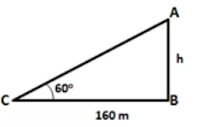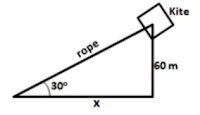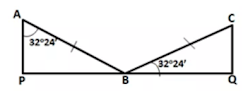# ICSE Solutions for Chapter 22 Heights and Distances Class 10 Mathematics

Question 1: The height of a tree is 3 times the length of its shadow. Find the angle of elevation of the sun.

Solution 1:Let the length of the shadow of the tree be x m.
∴ Height of the tree √3 x m
If θ  is the angle of elevation of the sun, then
tan θ = √3x/x = √3 = tan 60°
∴ θ = 60°

Question 2: The angle of elevation of the top of a tower from a point on the ground and at a distance of 160 m from its foot, is found to be 60°. Find the height of the tower.

Solution 2:Let the height of the tower be h m.
Given that angle of elevation is 60°
tan 60° = h/160
⇒ √3 = h/160
∴ h = 160√3 = 277.12 m
So, height of the tower is 277.12 m

Question 3: A ladder is placed along a wall such that its upper end is resting against a vertical wall. The foot of the ladder is 2.4 m from the wall and the ladder is making an angle of 68° with the ground. Find the height, upto which the ladder reaches.

Solution 3:Let the height upto which the ladder reaches be h m.
Given that angle of elevation is 68°
tan 68˚ = h/2.4
⇒ 2.475 = h/2.4
∴ h = 2.475 x 2.4 = 5.94 m
So, the ladder reaches upto a height of 5.94 m.

Question 4: Two persons are standing on the opposite sides of a tower. They observe the angles of elevation of the top of the tower to be 30° and 38° respectively. Find the distance between them, if the height of the tower is 50 m.

Solution 4:Let one person A be at a distance x and the second person B be at a distance of y from the foot of the tower.
Given that angle of elevation of A is 30°
tan 30° = 50/x
⇒ 1/√3 = 50/x
∴ x = 50/√3 = 86.60 m
The angle of elevation of B is 38˚
tan 38˚ = 50/y
⇒ 0.7813 = 50/y
∴ y ≈ 64m
So, distance between A and B is x + y = 150.6 m

Question 5: A kite is attached to a string. Find the length of the string, when the height of the kite is 60 m and the string makes an angle 30° with the ground.

Solution 5:Let the length of the rope be x m.
Now,
sin 30° = 60/x
⇒ ½ = 60/x
∴ x = 120 m
So, the length of the rope is 120m.

Question 6: A boy, 1.6 m tall, is 20 m away from a tower and observes the angle of elevation of the top of the tower to be (i) 45° (ii) 60°. Find the height of the tower in each case.

Solution 6: Let the height of the tower be h m.
(i) Here θ = 45°
∴ tan 45˚ = (h - 1.6)/20
⇒ 1 = (h - 21.6)/20
∴ h = 21.6 m
So, height of the tower is 21.6 m.

(ii) Here θ = 60°
∴ tan 60° = (h – 1.6)/20
⇒ √3 = (h – 1.6)/20
∴ h = 20 x 1.732 + 1.6  = 36.24 m
So, height of the tower is 36.24 m.

Question 7: The upper part of a tree, broken over by the wind, makes an angle of 45° with the ground and the distance from the root to the point where the top of the tree touches the ground is 15 m. What was the height of the tree before it was broken?

Solution 7: Let the height of the tree after breaking be h m.
Here, θ = 45°
∴ tan 45˚ = h/15
⇒ 1 = h/15
∴ h = 15 m
Now, length of the tree broken by the wind = 15/sin 45˚ = 15√2 = 21.21 m
So, height of the tree before it was broken is (15 + 21.21) m = 36.21 m.

Question 8: The angle of elevation of the top of an unfinished tower at a point distance 80 m from its base is 30°. How much higher must the tower be raised so that its angle of elevation at the same point may be 60°?

Solution 8:Let AB be the unfinished tower and C be the top of the tower when finished. Let P be a point 80 m from the foot A.
In ΔBAP
tan 30° = AB/AP
⇒ 1/√3 = AB/80
⇒ AB = 80/√3 = 46.19 m

In ΔCAP,
tan 60° = AC/AP
⇒ √3 = AC/80
⇒ AC = 80√3 = 138.56 m
Therefore, the tower must be raised by (138.56 - 46.19)m = 92.37m

Question 9: At a particular time, when the sun's altitude is 30°, the length of the shadow of a vertical tower is 45 m. Calculate
(i) the length of the tower.
(ii) the length of the shadow of the same tower, when the sun's altitude is
(a) 45° (b) 60°

Solution 9: Let the length of the tower be h m.
(i) Here θ = 30°
tan 30° = h/45
⇒ 1/√3 = h/45
⇒ h = 25.98 m
Hence the length of the tower is 25.98 m.

(ii) Let the length of the shadow be x m.
(a) Here, θ = 45˚
tan 45˚ = 25.98/x
⇒ 1 = 25.98/x
⇒ x = 25.98 m
Hence the length of the shadow is 25.98 m

(b) Here, θ = 60°
tan 60° = 25.98/x
⇒ √3 = 25.98/x
⇒ x = 25.98/√3   = 15m
Hence the length of the shadow is 15 m.

Question 10: Two vertical poles are on either side of a road. A 30 m long ladder is placed between the two poles. When the ladder rests against one pole, it makes angle 32°24' with the pole and when it is turned to rest against another pole, it makes angle 32°24' with the road. Calculate the width of the road,

Solution 10:Let AB be the ladder and ∠ABP = 32°24;
∴ BP/AB = sin 32°24'
⇒ BP = 30×0.536 = 16.08
When rotated, let the ladder be AC and ∠CAQ = 32°24;
∴ BQ/BC = cos 32°24'
⇒ BQ = 30×0.844 = 25.32
Hence, width of the road = (16.08 + 25.32) = 41.4 m